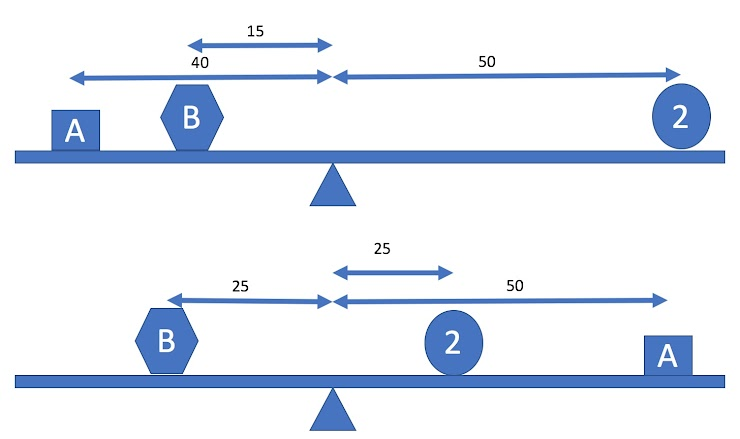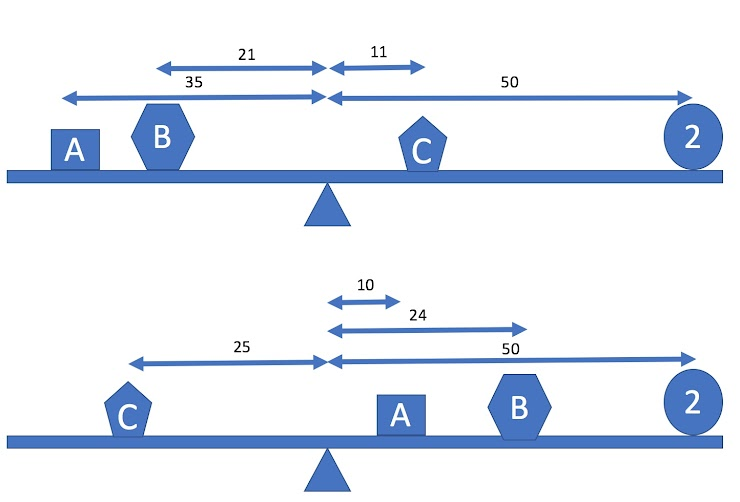# 01 In-Class Assignment: Welcome to Matrix Algebra with computational applications¶

What can you solve with $$Ax=b$$?Image from http://wikipedia.org/

from IPython.display import YouTubeVideo


## 1. Class Procedures¶

All in-class assignments are designed such that you can get started as soon as you show up in class. This is highly recommended. See how far you can get on your own and then you will be ready when it is time for questions. Here are the basic instructions.

### ✅ Step 1 - Get out your laptop¶

Feel free to grab one of the laptops in the classroom if you do not have your own.

### ✅ Step 2 - Create a Course Assignment Folder in your home directory¶

HINT Store all notebooks in the same folder on your computer. They will work better that way.

Download a copy of this notebook (ipynb file which tands for ipython notebook).

### ✅ Step 4 - Open this assignment in Jupyter¶

Open the downloaded file inside of jupyter either on your laptop or upload the file to an on-line server and open it there.

## 2. Introductions¶

This course appeals to students from many different backgrounds. Let us use this time to go around the room and introduce ourselves to each other.

## 3. Example:¶

Suppose that we have three objects on a balanced beam. Also suppose we know that one has a mass of 2 kg, and we want to find the two unknown masses. Experimentation with a (assume weightless) meter stick produces these two balances. (diagram not to scale)For the masses to balance we must have the sum of the moments on the left equal to the sum of the moments on the right, where the moment of an object is its mass times its distance from the balance point. That gives a system of two equations:

$40A + 15B = 50 \times 2$
$25B = 25 \times 2 + 50A$

Do This: Find a solution for the above systems of equations and place your solution in the following cell. Make sure you delete the instructional text in the cell first.

# Put your answer to the above question here


Do This: Using Python as a calculator, verify that the solution you have found is correct.

# Put your answer to the above question here


Do This: Now lets consider a system where we have three unknown masses instead of two. Experimentation with a meter stick produces the two balanced states shown below (diagram not to scale). Write the equations for this system.Do This: Find a solution to the second set of equations and report the mass for objects A, B and C.

# Put your answer to the above question here


Do This: Using Python as a calculator, verify that the solution you have found is correct.

# Put your answer to the above question here


## 4. Syllabus, Schedule and other Procedures¶

Let us now use the time to review the course Syllabus, schedule and other procedures.